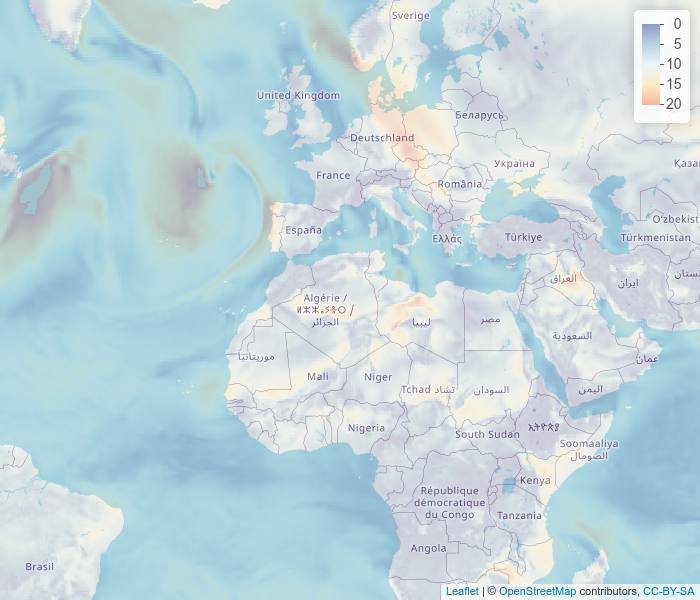# Example application

In some cases it is desirable to calculate the tiles not from a fixed grid but rather dynamically. Example are external data sources such as databases or storage systems. Also in some cases you would like to be able to dynamically calculate derivatives from grids.

This is a small example calculating wind speed on the fly from weather data on the fly. starsTileServer can do this as you can provide functions that calculate the grid on the fly. To do this there needs to be a function that takes a stars grid as the first argument and returns the same grid with values annotated. I have also added a function the determines the coloring of the grid.

In this case the data is extracted form a stars proxy object (this means the function is slower compared to all data being present in memory, but means not all data needs to be loaded. U an V are wind speeds in north south and east west direction. Speed is calculated using Pythagorean theorem. Much more complicated function can be developed but this just gives an idea. This is the function we will use the weather data file we also use in the other vignette. This file can also be downloaded directly from here https://surfdrive.surf.nl/files/index.php/s/XndqfCYJqkdjcFO/download.

windDirFun <- function(grd,
level = c("875", "900", "925"),
time = "2000-04-28 23:00:00") {
weather <- read_stars(options("tmpGridFile")[], proxy = T)
st_crs(weather) <- 4326
bbox <- st_bbox(
st_transform(
st_as_sf(grd),
st_crs(weather)
)
)
levelDim <- which(as.character(st_get_dimension_values(weather, "level")) == level)
timeDim <- which(as.character(st_get_dimension_values(weather, "time")) == time)
u <-
weather["u"] %>%
slice(level, levelDim) %>%
slice(time, timeDim)
), bbox), grd))
v <-
weather["v"] %>%
slice(level, levelDim) %>%
slice(time, timeDim)
), bbox), grd))
return(sqrt(u^2 + v^2))
}

We also create a color function to create a consistent color scale.


colFun <- function(x, alpha = 1, maxColor = 25) {
cfun <- leaflet::colorNumeric("RdYlBu", domain = c(-as.numeric(maxColor), 0))
paste0(
suppressWarnings(cfun(-x)),
as.character(as.raw(as.numeric(alpha) * 255))
)
}
attr(colFun, "colorType") <- "numeric"

To run the server we will again use a separate process with callr.

library(starsTileServer)
require(callr)

rp <- r_bg(args = list(tmpGridFile = tmpGridFile, windDirFun = windDirFun, colFun = colFun), function(tmpGridFile, windDirFun, colFun) {
require(sf)
require(stars)
require(dplyr)
options(tmpGridFile = tmpGridFile)
starsTileServer::starsTileServer$new(windDirFun, colorFun = colFun )$run(port = 5645, docs = T)
})

The following url can be used to explore the tile server when it is running: http://127.0.0.1:5645/__docs__/

Now we can plot a map, the url to the tiles here make it possible to select specific layers (e.g. the 900 mb level and the 2000-04-28 23:00:00 timestamp in this case).

require(leaflet)
require(leaflet.extras)
require(magrittr)
m <- leaflet() %>%
enableTileCaching() %>%
"http://127.0.0.1:5645/map/{z}/{x}/{y}?level=900&alpha=.2&time=2000-04-28 23:00:00",
options = tileOptions(useCache = TRUE, crossOrigin = TRUE)
) %>%
setView(zoom = 3, lat = 30, lng = 5)Before finishing it is important to close the server that is running in a separate process.

message(rp$read_error()) #> Loading required package: sf #> Linking to GEOS 3.8.0, GDAL 3.0.4, PROJ 6.3.1; sf_use_s2() is TRUE #> Loading required package: stars #> Loading required package: abind #> Loading required package: dplyr #> #> Attaching package: ‘dplyr’ #> #> The following objects are masked from ‘package:stats’: #> #> filter, lag #> #> The following objects are masked from ‘package:base’: #> #> intersect, setdiff, setequal, union #> #> Running plumber API at http://127.0.0.1:5645 #> Running swagger Docs at http://127.0.0.1:5645/__docs__/ message(rp$read_output())
#> t, z, u, v,
#> t, z, u, v,
#> t, z, u, v,
#> t, z, u, v,
#> t, z, u, v,
#> t, z, u, v,
#> t, z, u, v,
#> t, z, u, v,
#> t, z, u, v,
#> t, z, u, v,
#> t, z, u, v,
#> t, z, u, v,
rp\$finalize()